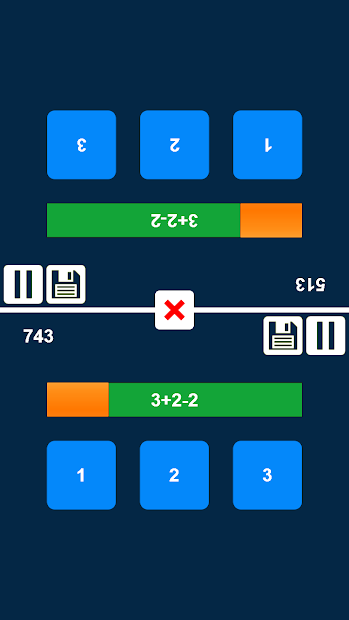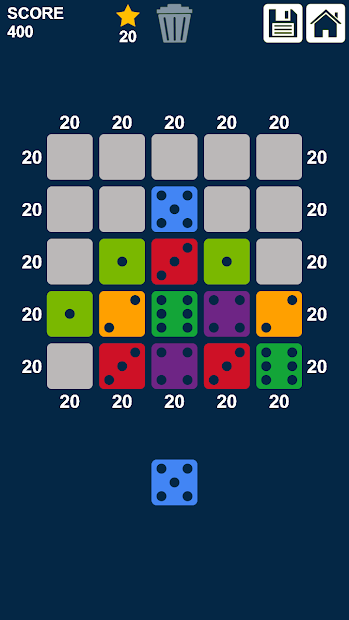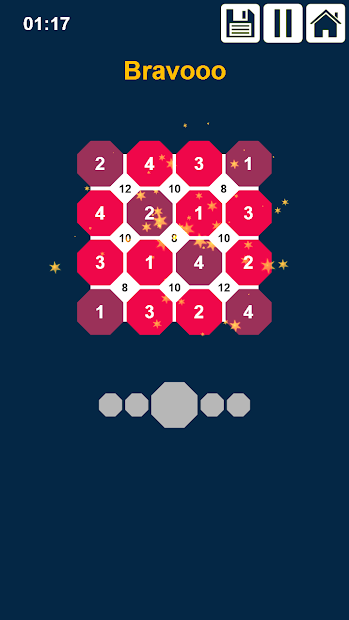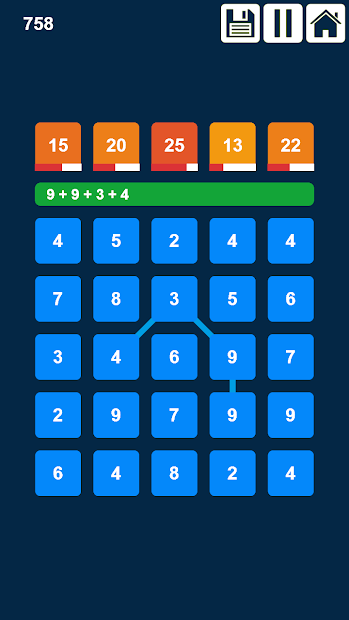11-22-2019 08:34 PM
1. This is a collection of 11 arithmetic games to test your mental math skills. Best math practice for the whole family kids and adults.

Play Store URL: Arithmetic Math Games CollectionList of games:
Arithmetic Addition: Connect 3 or more numbers so that their sum is equal to one of the target numbers.

Arithmetic Multiplication: Connect 3 or more numbers so that the result of their multiplication is equal to one of the target numbers.

Arithmetic Division: Connect 3 or more numbers so that the result of their division is equal to one of the target numbers.

Arithmetic Subtraction: Connect 3 or more numbers so that the result of their subtraction is equal to one of the target numbers.

1 + 2 = 3: It’s a simple math addition and subtraction game where its equations consist of the numbers 1, 2 and 3 and its result is also 1, 2 or 3. Tap the correct answer.

1 + 2 = 3 Duel: It’s a simple math addition and subtraction game where 2 persons are competing on the same device. The score will be counted for the person who touches the correct answer first.

Arithmetic Challenge: It’s a math addition, subtraction, division and multiplication game where its equations consist of the numbers between 1 and 99 and its result is also a number between 1 and 99. Tap the correct answer.

Arithmetic Duel: t’s a math addition, subtraction, division and multiplication game where its equations consist of the numbers between 1 and 99 and its result is also a number between 1 and 99. 2 persons are competing on the same device. The score will be counted for the person who touches the correct answer first.

Number Junctions: Sudoku Addition: Fill in the empty circles (octagons) so that the number between the four adjacent circles is the result of their sum. But be careful a number can't be used more than once in any column or row like in Sudoku.

Number Junctions: Sudoku Multiplication: Fill in the empty circles (octagons) so that the number between the four adjacent circles is the result of their multiplication. But be careful a number can't be used more than once in any column or row like in Sudoku.

Dominoes Make 20: Drag and place dominoes on the grid so that the sum of dots of the non-empty rows or columns = 20

I hope you like it.
11-22-2019 08:34 PM
LINK TO POST COPIED TO CLIPBOARD